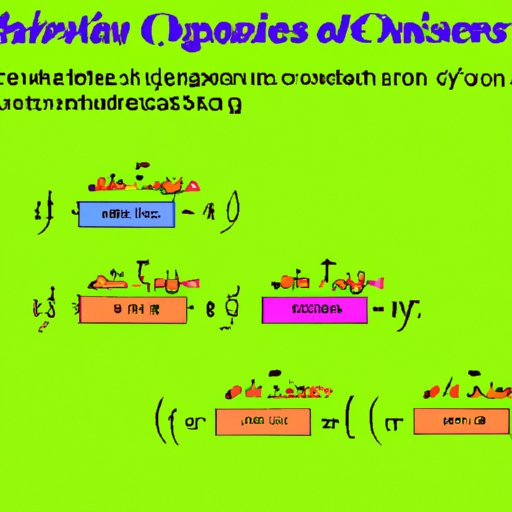# How to Solve Optimization Problems Using Calculus## Introduction

Optimization problems are mathematical problems that involve finding the best solution from among a set of possible solutions. The goal is to maximize or minimize a certain quantity, such as profit or cost. Calculus is a branch of mathematics that deals with rates of change and can be used to solve optimization problems. In this article, we will explore the fundamentals of calculus in solving optimization problems, provide examples of optimization problems and their solutions using calculus, discuss constraints when solving optimization problems, present different methods of optimization, and offer tips on approaching an optimization problem from a calculus perspective.

## Fundamentals of Calculus in Solving Optimization Problems

Calculus is essential in solving optimization problems because it allows us to find the maximum or minimum value of a function. This is done by taking derivatives and integrals. A derivative is the rate of change of a function at a given point, while an integral is the area under the graph of a function. Differentiation rules, such as the chain rule and the product rule, can be used to find the derivative of a function, which is necessary for finding the maximum or minimum value of a function.

## Examples of Optimization Problems and Their Solutions Using Calculus

Let’s look at two examples of optimization problems and their solutions using calculus. The first example involves finding the maximum value of a function. For this problem, we must first identify the function and its domain. Then, we must take the derivative of the function to determine the critical points (points where the derivative is equal to zero). Finally, we must evaluate the function at the critical points to determine the maximum value.

The second example involves finding the minimum value of a function. Again, we must first identify the function and its domain. Next, we must take the derivative of the function to determine the critical points. We then evaluate the function at the critical points to determine the minimum value.

## Understanding Constraints When Solving Optimization Problems

When solving optimization problems, it is important to understand the types of constraints that may be present. Constraints are restrictions that limit the range of values that can be used in the solution. Examples of constraints include time limits, budget limits, and resource availability. To account for constraints in the solution process, we must modify the objective function and/or add additional constraints.

## Different Methods of Optimization

There are several different methods that can be used to solve optimization problems. These methods include linear programming, dynamic programming, and nonlinear programming. Linear programming involves finding the optimal solution from a set of linear equations. Dynamic programming involves breaking down a complex problem into simpler subproblems. Nonlinear programming involves finding the optimal solution from a set of nonlinear equations.

## Resources for Further Learning about Calculus and Optimization

If you are interested in learning more about calculus and optimization, there are many resources available. Textbooks such as Calculus: An Applied Approach by Ron Larson and Bruce Edwards can provide an in-depth introduction to calculus and its applications. Online tutorials such as Khan Academy can also be helpful in understanding the fundamentals of calculus. Additionally, there are many websites devoted specifically to optimization problems and their solutions.

## Tips on Approaching an Optimization Problem from a Calculus Perspective

When approaching an optimization problem from a calculus perspective, there are several tips that can be useful. First, break down the problem into smaller pieces. This will help you identify the relevant variables and determine the best approach. Second, identify any constraints that may be present. This will help you determine the range of values that can be used in the solution. Finally, utilize different techniques such as differentiation, integration, linear programming, dynamic programming, and nonlinear programming to find the optimal solution.

## Conclusion

In conclusion, optimization problems can be solved using calculus. Calculus involves taking derivatives and integrals, which can be used to find the maximum or minimum value of a function. Examples of optimization problems and their solutions using calculus were provided, as well as an overview of constraints and different methods of optimization. Additionally, resources for further learning about calculus and optimization were discussed, as well as tips on approaching an optimization problem from a calculus perspective. With these tools, you should be able to tackle optimization problems with confidence.#### By Happy Sharer

Hi, I'm Happy Sharer and I love sharing interesting and useful knowledge with others. I have a passion for learning and enjoy explaining complex concepts in a simple way.